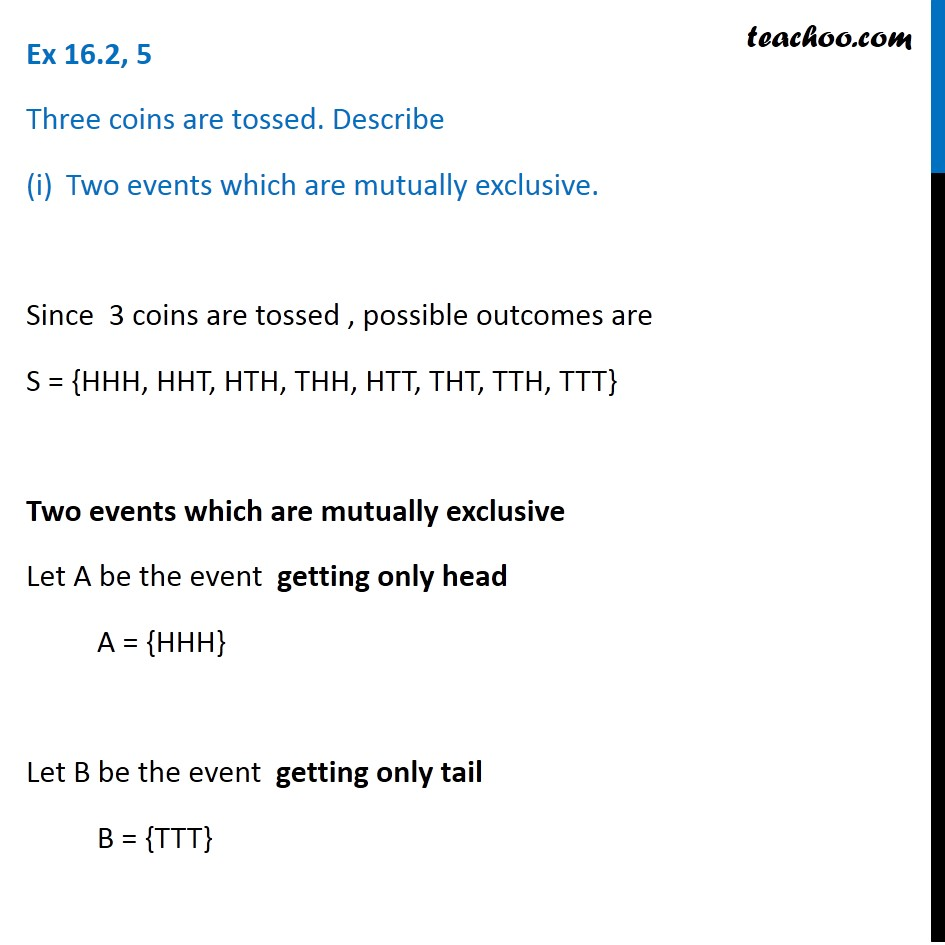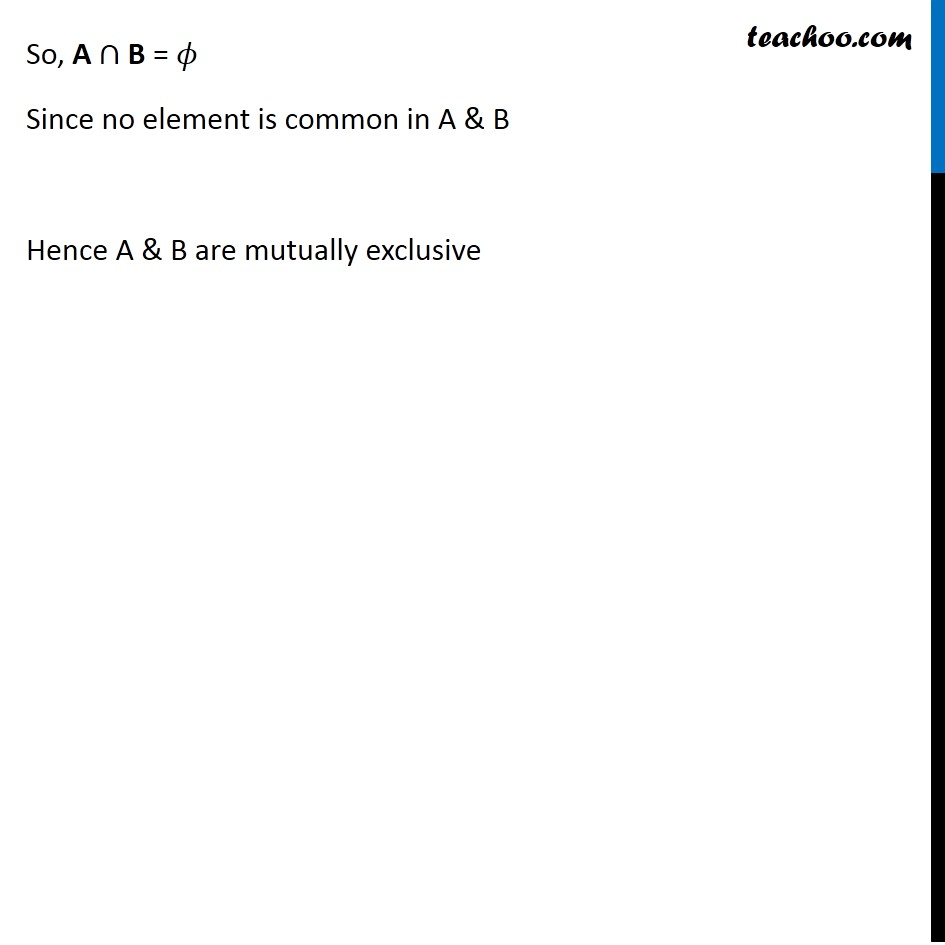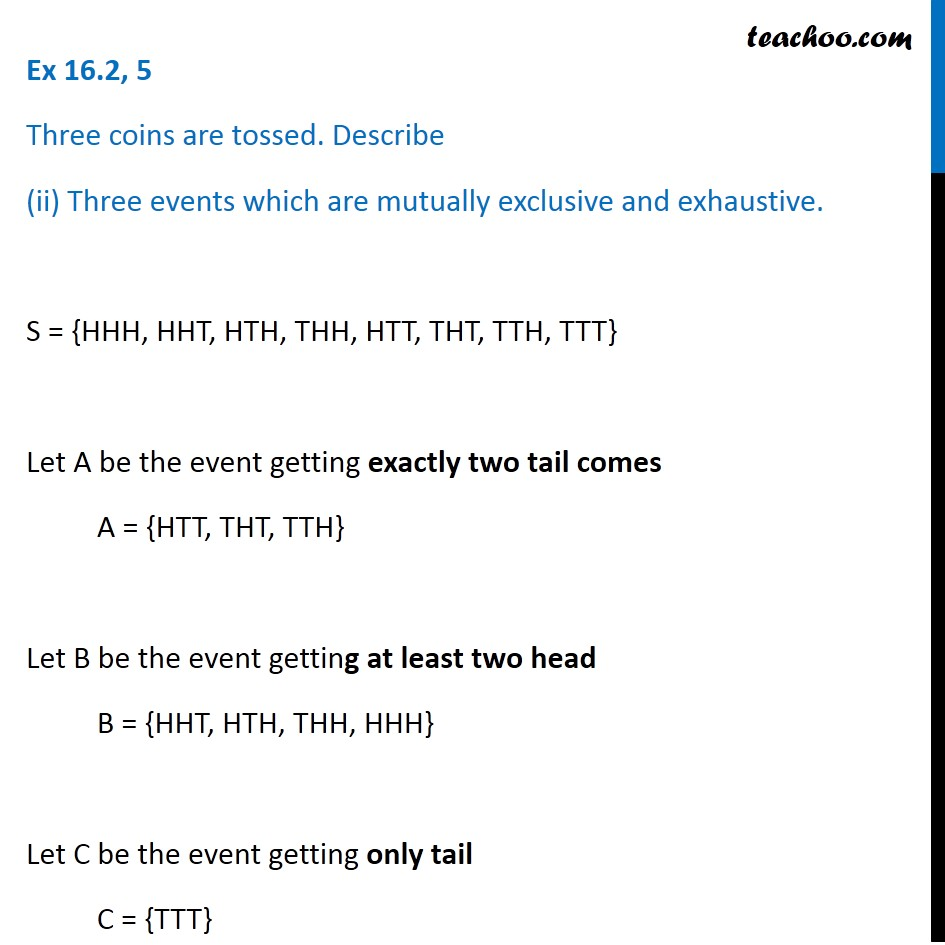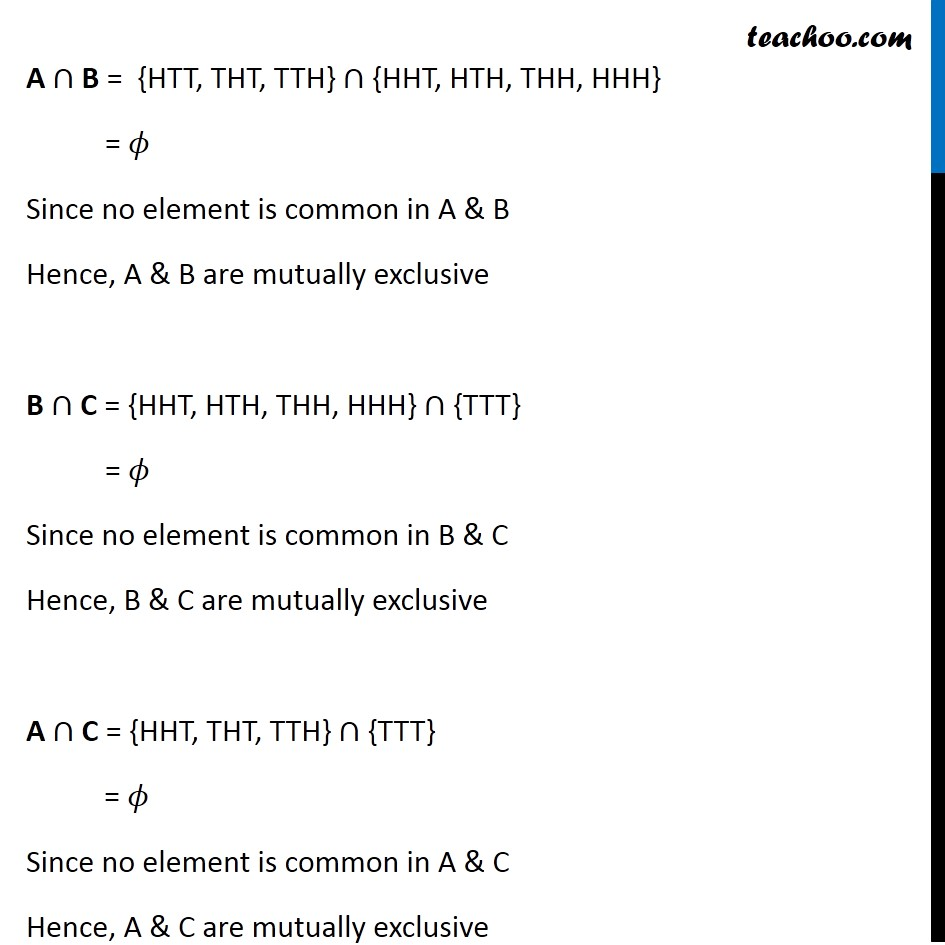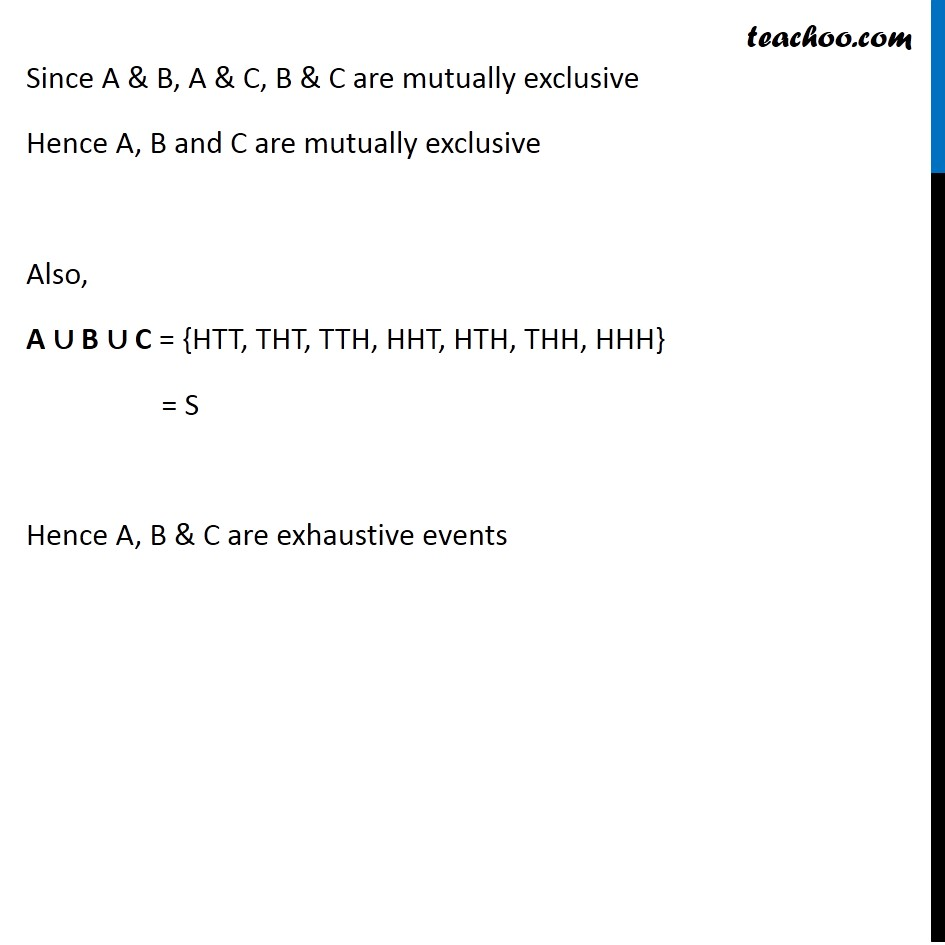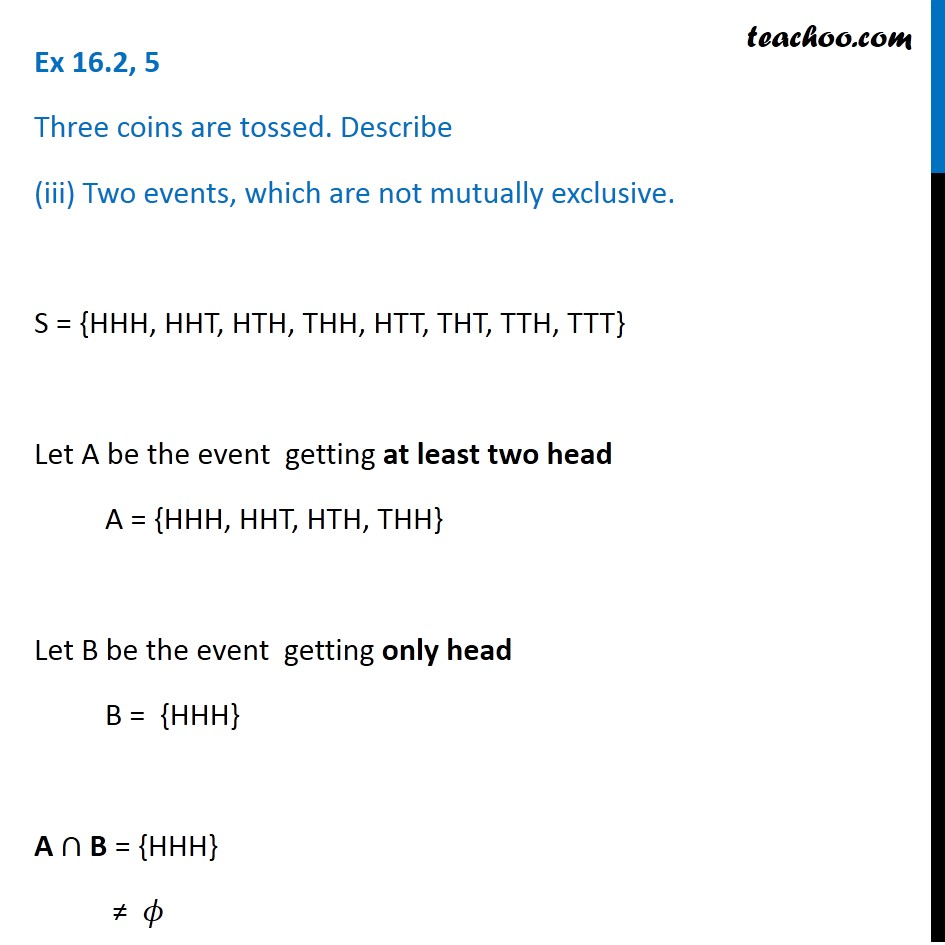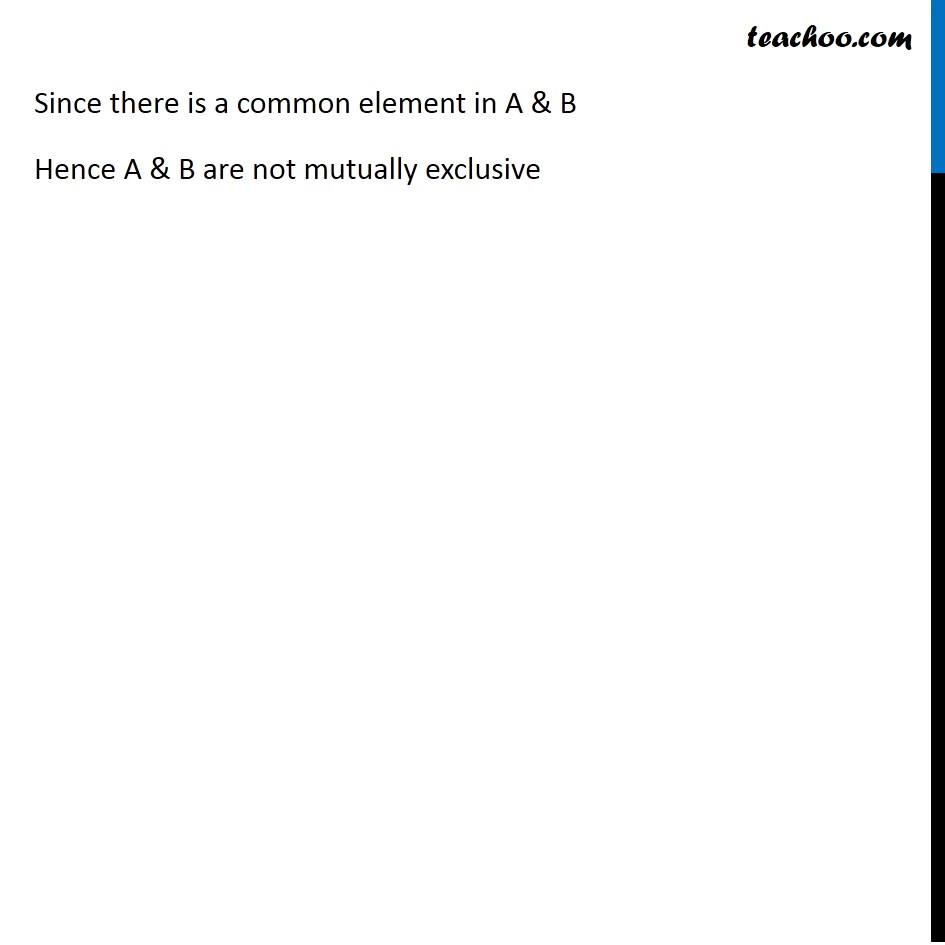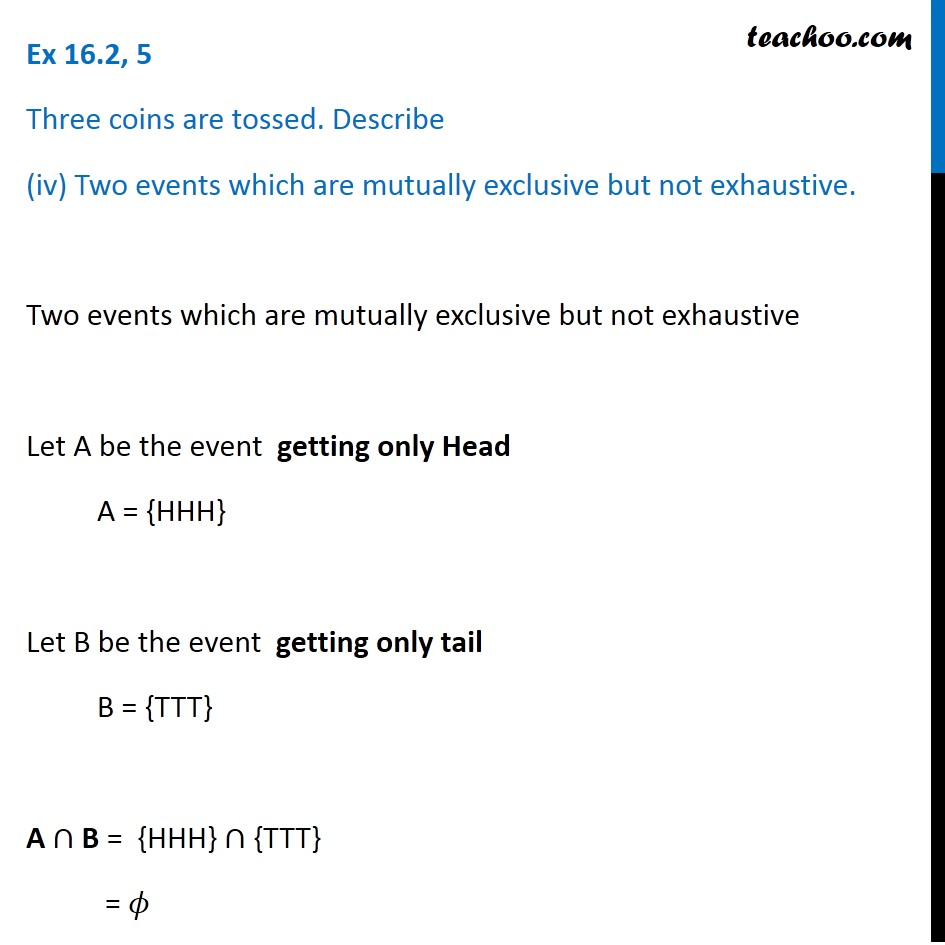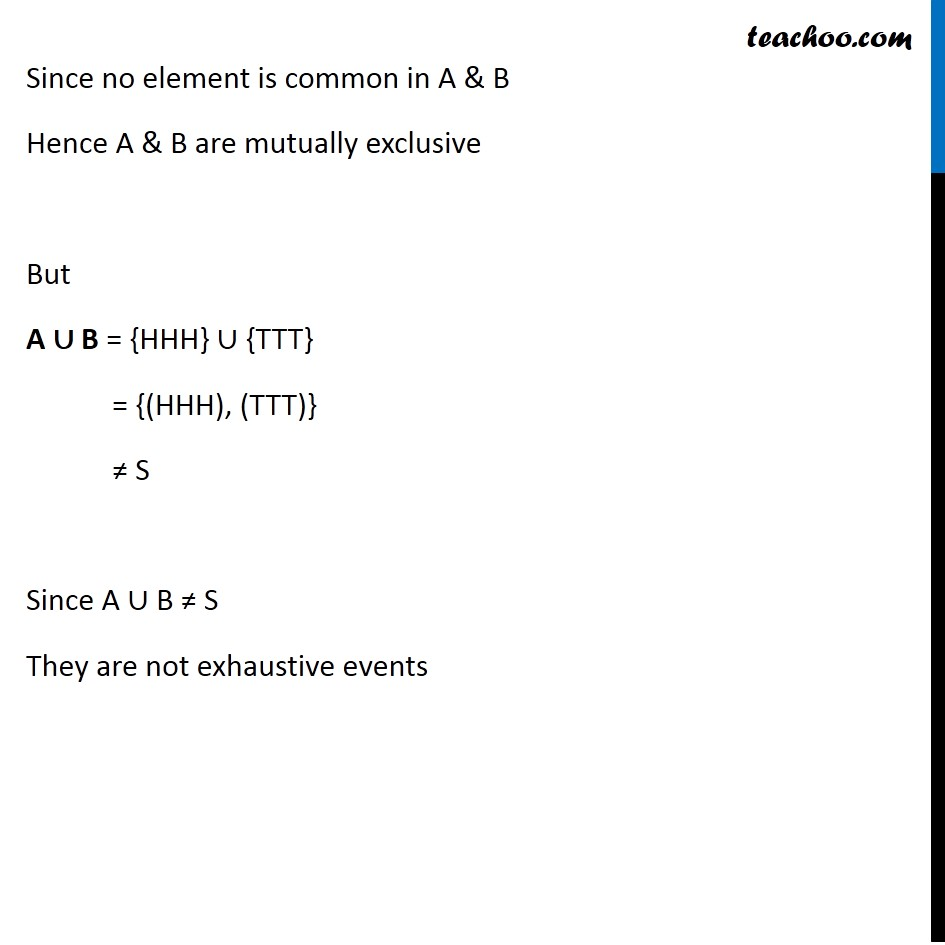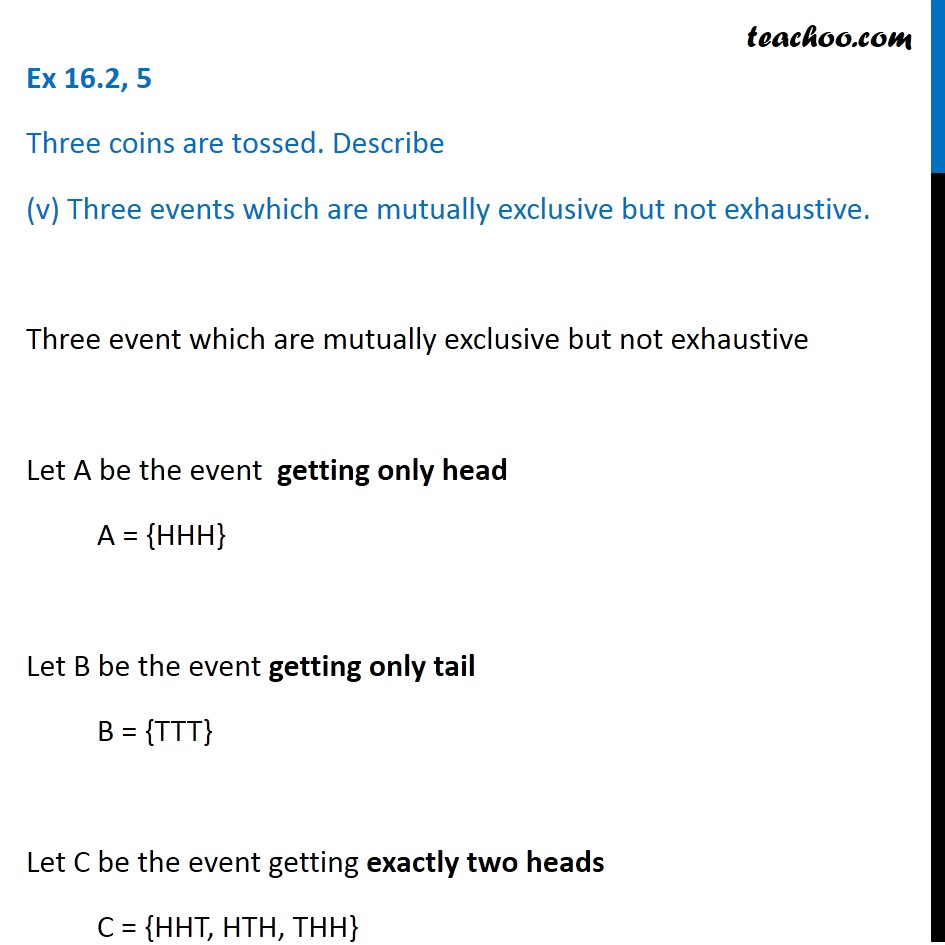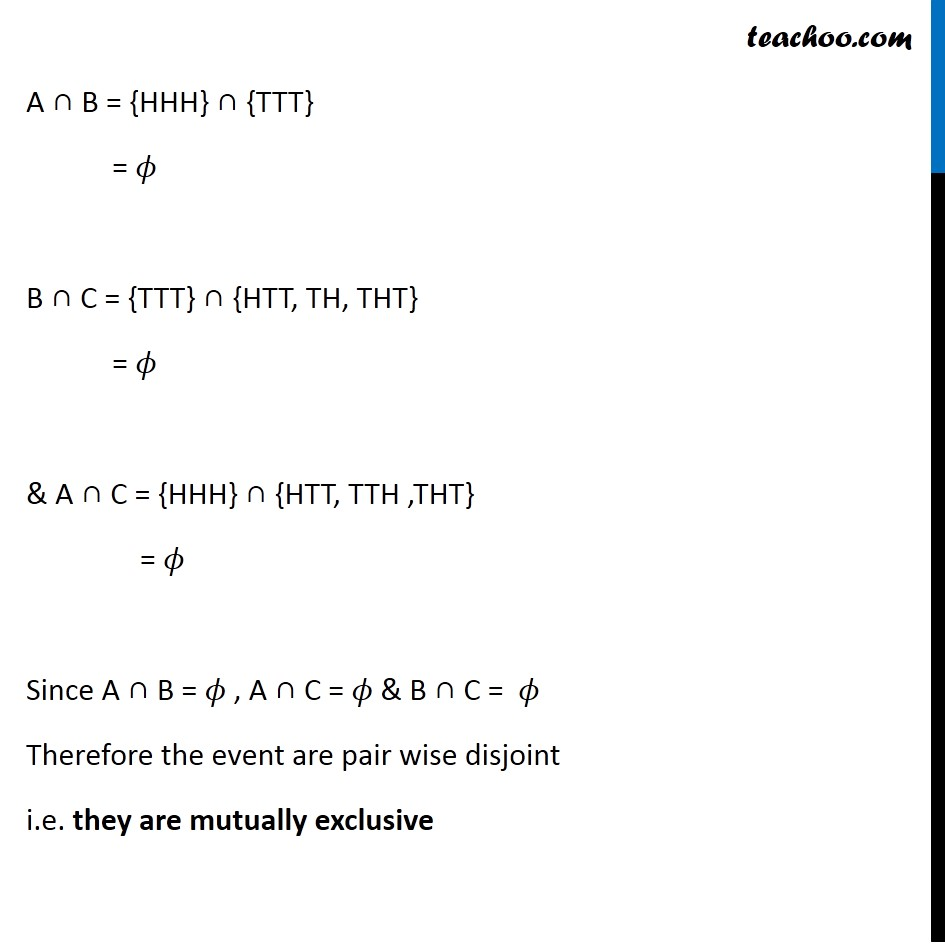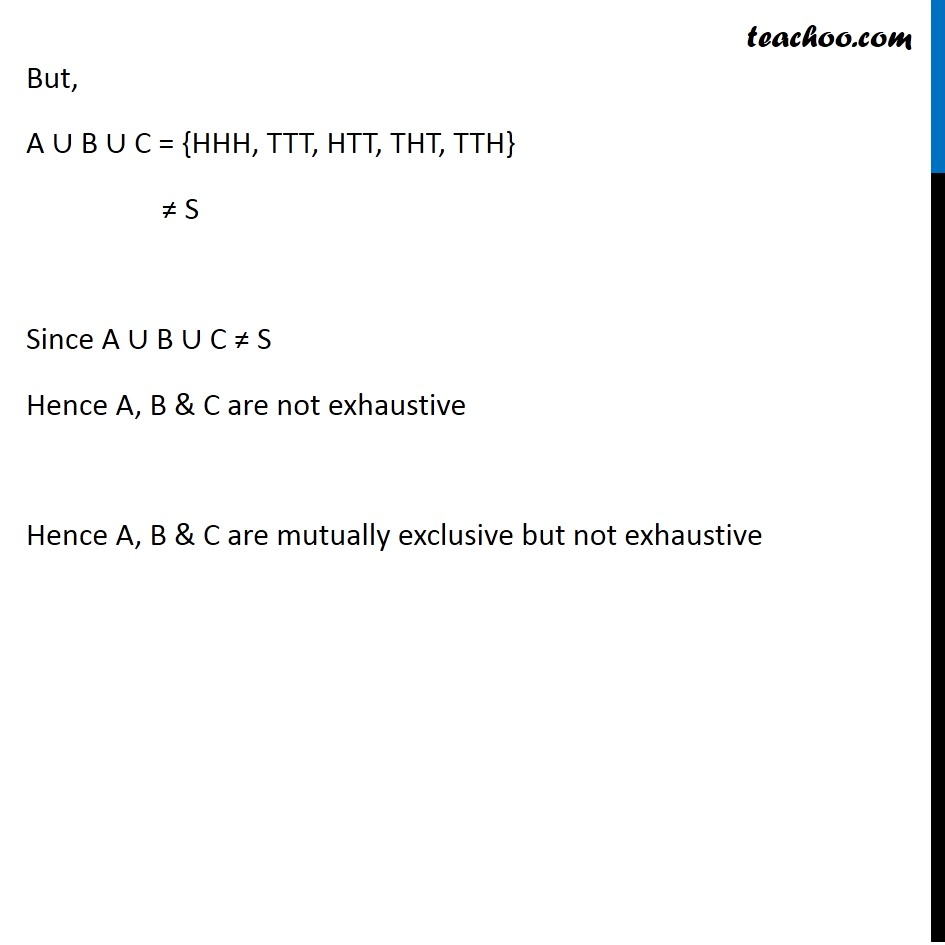Subscribe to our Youtube Channel - https://you.tube/teachoo

1. Chapter 16 Class 11 Probability
2. Serial order wise
3. Ex 16.2

Transcript

Ex 16.2, 5 Three coins are tossed. Describe Two events which are mutually exclusive. Since 3 coins are tossed , possible outcomes are S = {HHH, HHT, HTH, THH, HTT, THT, TTH, TTT} Two events which are mutually exclusive Let A be the event getting only head A = {HHH} Let B be the event getting only tail B = {TTT} So, A ∩ B = 𝜙 Since no element is common in A & B Hence A & B are mutually exclusive Ex 16.2, 5 Three coins are tossed. Describe (ii) Three events which are mutually exclusive and exhaustive. S = {HHH, HHT, HTH, THH, HTT, THT, TTH, TTT} Let A be the event getting exactly two tail comes A = {HTT, THT, TTH} Let B be the event getting at least two head B = {HHT, HTH, THH, HHH} Let C be the event getting only tail C = {TTT} A ∩ B = {HTT, THT, TTH} ∩ {HHT, HTH, THH, HHH} = 𝜙 Since no element is common in A & B Hence, A & B are mutually exclusive B ∩ C = {HHT, HTH, THH, HHH} ∩ {TTT} = 𝜙 Since no element is common in B & C Hence, B & C are mutually exclusive A ∩ C = {HHT, THT, TTH} ∩ {TTT} = 𝜙 Since no element is common in A & C Hence, A & C are mutually exclusive Since A & B, A & C, B & C are mutually exclusive Hence A, B and C are mutually exclusive Also, A ∪ B ∪ C = {HTT, THT, TTH, HHT, HTH, THH, HHH} = S Hence A, B & C are exhaustive events Ex 16.2, 5 Three coins are tossed. Describe (iii) Two events, which are not mutually exclusive. S = {HHH, HHT, HTH, THH, HTT, THT, TTH, TTT} Let A be the event getting at least two head A = {HHH, HHT, HTH, THH} Let B be the event getting only head B = {HHH} A ∩ B = {HHH} ≠ 𝜙 Since there is a common element in A & B Hence A & B are not mutually exclusive Ex 16.2, 5 Three coins are tossed. Describe (iv) Two events which are mutually exclusive but not exhaustive. Two events which are mutually exclusive but not exhaustive Let A be the event getting only Head A = {HHH} Let B be the event getting only tail B = {TTT} A ∩ B = {HHH} ∩ {TTT} = 𝜙 Since no element is common in A & B Hence A & B are mutually exclusive But A ∪ B = {HHH} ∪ {TTT} = {(HHH), (TTT)} ≠ S Since A ∪ B ≠ S They are not exhaustive events Ex 16.2, 5 Three coins are tossed. Describe (v) Three events which are mutually exclusive but not exhaustive. Three event which are mutually exclusive but not exhaustive Let A be the event getting only head A = {HHH} Let B be the event getting only tail B = {TTT} Let C be the event getting exactly two heads C = {HHT, HTH, THH} A ∩ B = {HHH} ∩ {TTT} = 𝜙 B ∩ C = {TTT} ∩ {HTT, TH, THT} = 𝜙 & A ∩ C = {HHH} ∩ {HTT, TTH ,THT} = 𝜙 Since A ∩ B = 𝜙 , A ∩ C = 𝜙 & B ∩ C = 𝜙 Therefore the event are pair wise disjoint i.e. they are mutually exclusive But, A ∪ B ∪ C = {HHH, TTT, HTT, THT, TTH} ≠ S Since A ∪ B ∪ C ≠ S Hence A, B & C are not exhaustive Hence A, B & C are mutually exclusive but not exhaustive

Ex 16.2